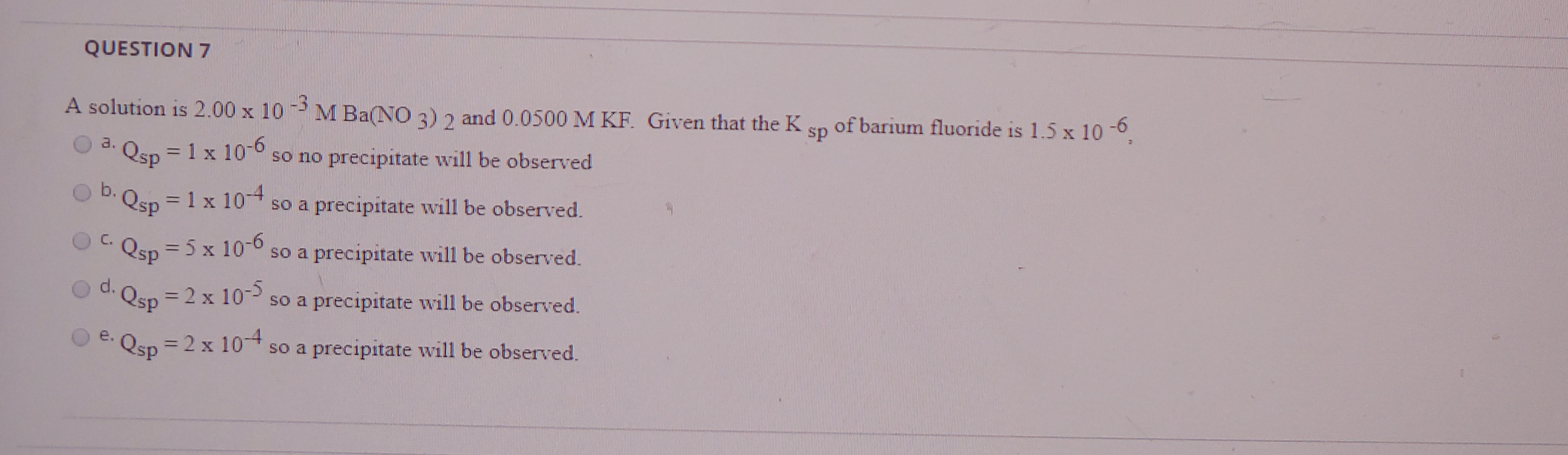# A solution is 2.00 x 10-3 M Ba(NO3)2 and 0.0500 M KF. Given that the Ksp of barium fluoride is 1.5 x 10-6. a. Qsp = 1 x 10^-6, so no precipitate will be observed b. Qsp = 1x 10^-4, so a precipitate will be observed c. Qsp = 5 x 10^-6, so a precipitate will be observed d. Qsp = 2 x 10^-5, so a precipitate will be observed e. Qsp = 2 x 10^-4, so a precipitate will be observed I need help understanding the steps to this solubility and complex ion equilibria question